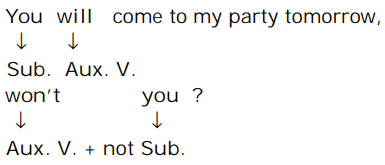## COMMON ERRORS

#### COMMON ERRORS

1. Solve the question

1. Structure of Question Tag in Future Tense with will is as follows :Contraction of will not is won’t.
Hence, won’t you is the right usage.

##### Correct Option: C

Structure of Question Tag in Future Tense with will is as follows :Contraction of will not is won’t.
Hence, won’t you is the right usage.

1. Solve the question

1. Here, money is an uncountable Noun. Hence,Singular Verb i.e. was spent is the right usage

##### Correct Option: B

Here, money is an uncountable Noun. Hence,Singular Verb i.e. was spent is the right usage

1. Solve the question

1. Rustam and I is the Sub. of the sentence, so the Nominative Pro. is used for the sentence for making it grammatically correct. Hence, I saw is the right usage.

##### Correct Option: B

Rustam and I is the Sub. of the sentence, so the Nominative Pro. is used for the sentence for making it grammatically correct. Hence, I saw is the right usage.

1. Solve the question

1. No error.

##### Correct Option: D

No error.

1. Solve the question

1. It is a past event. Hence, Past Simple i.e. I saw is the right usage

##### Correct Option: A

It is a past event. Hence, Past Simple i.e. I saw is the right usage